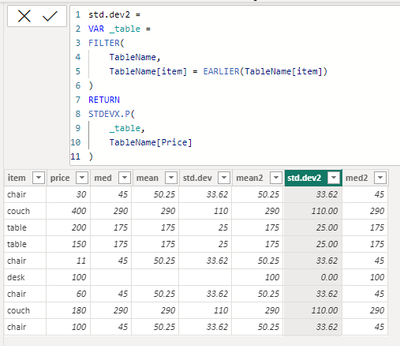cancel
Showing results for
Did you mean:Anonymous
Not applicable

## Grouped norm dist

Hi all,

I'm trying to make a grouped norm.dist calculation but can't yet figure it out. Below an example of my dataset (item, price):

 chair 30 couch 400 table 200 table 150 chair 11 desk 100 chair 60 couch 180 chair 100

If I would do a normal norm.dist calculation it wouldn't do any good since there are different products. That's why I want to group the calculation based on the product column. Each item should be calculated on it's own median and mean prices, and not on all the prices. It's btw big data with around 1K different items.

1 ACCEPTED SOLUTIONCommunity Champion

Aha, @Anonymous  try to add three columns like:

``````mean2 =
VAR _table =
FILTER(
TableName,
TableName[item] = EARLIER(TableName[item])
)
RETURN
AVERAGEX(
_table,
TableName[Price]
)

med2 =
VAR _table =
FILTER(
TableName,
TableName[item] = EARLIER(TableName[item])
)
RETURN
CONVERT(
MEDIANX(
_table,
TableName[Price]
),
INTEGER
)

std.dev2 =
VAR _table =
FILTER(
TableName,
TableName[item] = EARLIER(TableName[item])
)
RETURN
STDEVX.P(
_table,
TableName[Price]
)``````

it worked like:6 REPLIES 6Community Champion

hi @Anonymous

try to plot a table visual with the product column and measures like:

mean = MEAN(TableName[Price])
median = MEDIAN(TableName[Price])

Or?Anonymous
Not applicable

I would like to have the data in a calculated column, so that won't work. Besides that I dont want the distribution over all the prices but the distribution for each product within it's own prices.Community Champion

hi @Anonymous

try to create a calculated table like:

Table =
VALUES(TableName[Product]),
"mean",
CALCULATE(MEAN(TableName[Price])),
"median",
CALCULATE(MEDIAN(TableName[Price]))
)Anonymous
Not applicable

The problem is that it calculates everything on all the data, while you want to know the mean/median/stddev based on the items prices. Example;

 item price stddev chair 30 A couch 400 B table 200 C table 150 C chair 11 A desk 100 D chair 60 A couch 180 B chair 100 AAnonymous
Not applicable

The problem is that it calculates it over all the data ... I want to group the values based on the items. Example:

 item price med mean std.dev chair 30 45 50.25 33.62 couch 400 290 290 110 table 200 175 175 25 table 150 175 175 25 chair 11 45 50.25 33.62 desk 100 null null null chair 60 45 50.25 33.62 couch 180 290 290 110 chair 100 45 50.25 33.62

The endgoal is to filter out outliers automatically within the report.Community Champion

Aha, @Anonymous  try to add three columns like:

``````mean2 =
VAR _table =
FILTER(
TableName,
TableName[item] = EARLIER(TableName[item])
)
RETURN
AVERAGEX(
_table,
TableName[Price]
)

med2 =
VAR _table =
FILTER(
TableName,
TableName[item] = EARLIER(TableName[item])
)
RETURN
CONVERT(
MEDIANX(
_table,
TableName[Price]
),
INTEGER
)

std.dev2 =
VAR _table =
FILTER(
TableName,
TableName[item] = EARLIER(TableName[item])
)
RETURN
STDEVX.P(
_table,
TableName[Price]
)``````

it worked like: